×
Log in to StudySoup
Get Full Access to UH - MATH 2311 - Class Notes - Week 1
Join StudySoup for FREE
Get Full Access to UH - MATH 2311 - Class Notes - Week 1

Already have an account? Login here
×
Reset your password

UH / Math / MATH 2311 / characteristics that start with m

# characteristics that start with m Description

##### Uploaded: 02/11/2017
12 Pages 160 Views 1 Unlocks
Reviews

Chapter 1: Exploring Univariate Data Section 1.1: Types of Data  ∙ Relevance of statistics - Statistics is used to gather and analyze data for any discipline  - Statistics is used to analyze surveys ∙ What is Statistics? - Statistics is used to make intelligent decisions in a world full of  uncertainty  “A knowledge of statistics provides the necessary tool to  differentiate between sound statistics conclusions and  questionable conclusions.” - Statistics is the science of collecting, organizing, and interpreting  numerical facts which we call data ∙ What is “Data”? - Statistics is the science of collecting, organizing and interpreting  numerical facts which we call data  The facts and figures collected, analyzed, and summarized for presentation and interpretation  Amount of your last purchase at a grocery store   The number of times that you access a certain website  Your name ∙ Types of Data:  - Population Data is everything or everyone we want information  about  It is a set of data that consists of all possible values pertaining to a certain set of observations or an investigation - Sample Data is a subset of the population that we have information  from  It is just a small section of the population taken for the  purpose of investigation ∙ Examples of Types of Data - Identify the population and the sample for each of the following:  University of Houston is interested in how many students buy  used books as opposed to new ones. They randomly choose  100 students at the student center to interview. o Population – All UH Students o Sample – 100 Students Samples  An elementary school is creating a new lunch menu. They  send questionnaires to students with last names that begin  with the letter M through R.  o Population – All students at this school o Sample – Students with last names that start with M  through R∙ A variable is a characteristic of an individual that can assume more  than one value - Variables can be classified as categorical (qualitative) or  quantitative (numeric).  Categorical variables – describe qualities or characteristics  that data may have o They usually represent a “type of something” such as a  type of car.   Quantitative variables – are measurements  o These will be numeric values ∙ Quantitative variables can be classified as either discrete or  continuous - Discrete quantitative variables – a countable set of values   For example: the number of lives given in a single play of a  video game - Continuous quantitative variables – data that can take on any  values within some interval   For example: the amount of time you wait in line at the  drivers license office ∙ Example: Classify the following variables as categorical or quantitative. - If quantitative, state whether the variable is discrete or continuous.  dPolitical preference o Categorical   Number of siblings o Quantitative - Discrete  Blood type o Categorical   Height of men on a professional basketball team  o Quantitative – Continuous   Time it takes to be on hold when calling the IRS at tax time o Quantitative – Continuous  Section 1.2: Mean and Median ∙ One question we want to answer about data is about its location,  particularly the location of its center. - Mean – is denoted with the Greek letter µ when referring to the  population mean and with the symbol ´x when referring to the  sample mean   Most common measure of center  Arithmetic average  We find the mean by adding up all the values and dividing by  how many.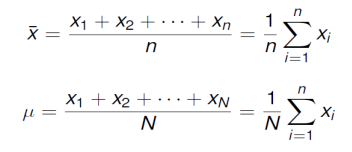 Where n is the size of the sample and N is the size of the  population  Symbols for mean: ´x vs µ - Median – M is the midpoint of a data set such that half of the  observations are smaller and the other half are larger  Arrange all observations in order of size, from smallest to  largest  Find the middle value of the arranged observations by  counting (n + 1)/2 from the bottom of the list o If the number of observations n is odd, the mean M is  the center observation in the ordered list.  o If the number of observations n is even, the median M is the mean of the two center observation in the ordered  list - Mode – is the numerical value that appears the most frequently   Mode is used as a description of center for categorical data  The data set can have one mode, two or more modes  A data set may not have any mode ∙ Examples:  - 1. Twelve babies spoke for the first time at the following ages (in  months):  8 9 10 11 12 13 15 15 18 20 20 26 a. What is the mean of the data? ´x = (8 + 9 + 10 + 11 + 12 + 13 + 15 + 15 + 18 + 20 + 20 +  26)/12 = 14.75 b. What is the median of the data? Median = (13 + 15)/2 = 14 c. What is the mode of the data? Bimodal modes are 15 and 20 - 2. Here are the weights (in pounds) of 20 steers on an experimental  feed diet: 174 142 131 145 175 150 176 151 110 162 133 163 135 178 178 154 166 146 156 167a. What is the mean of the data?  ´x = (174 + 142 + 131 + 145 + 175 + 150 + 176 + … + 167)/20  = 154.6 b. what is the mean of the data? 110, 131, 133, 135, 142, 145, 146, 150, 151, 154, 156, 162, 163, 166, 167, 174, 175, 176, 178, 178 Median = (154 + 156)/2 = 155 c. What is the mode of the data? Mode = 178 - 3. The test scores of a class of 20 students have a mean of 71.6 and the test scores of another class of 14 students have a mean of 78.4. Find the mean of the combined group.  Mean = sum/n Class 1: 71.6 = sum/20 sum = 20(71.6) = 1432 Class 2: 78.4 = sum/14 sum = 14(78.4) = 1097.6 Mean of combined classes = (1432 + 1097.6)/(20 + 14) = 74.4 - 4. Explain why the conclusion drawn is not valid: A businesswoman calculates that the median cost of the five  business trips that she took in a month is \$600 and concludes that  the total cost must have been \$3000. 1 2 3 4 5 6 \$600 If \$400 was mean the conclusion would be correct Section 1.3: Standard Deviation and Variance ∙ Another important question we want to answer about data is about its  spread or dispersion.  - Roughly speaking, the population standard deviation, σ, tells  the average distance that data values fall from the mean.  - The standard deviation is the square root of the population  variance, σ2.  - So, what is the variance?  - The variance is the average of the squared differences of the data  values from the mean. ∙ If N is the number of values in a population with mean μ , and xi  represents each individual value in the population, then the variance is found by: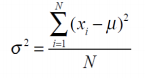∙ And the population standard deviation is σ = √σ2∙ Most of the time we are not working with the entire population.  - Instead, we are working with a sample.  Sample variance –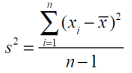 Sample standard deviation –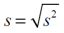∙ Example: - 1. A statistics teacher wants to decide whether or not to curve an  exam. From her class of 300 students, she chose a sample of 10  students and their grades were: 72, 88, 85, 81, 60, 54, 70, 72, 63, 43 Find the mean, variance and standard deviation for this sample. ´x = (72 + 88 + 85 + 81 + 60 + 54 + 70 + 72 + 63 + 43)/10 =  68.8 s2 = [(72 – 68.8)^2 + (88 – 68.8)^2 + (85 – 68.8)^2 + … + (43 –  68.8)^2]/(10 – 1) = ~199.7 s = √199.7 = ~14.13 - 2. Suppose the statistics teacher decides to curve the grades by  adding 10 points to each score. What is the new mean, variance and standard deviation? New mean: 78 (old mean + 10) or (68.8 + 10) New s2 = ~199.7 variance and standard deviation did not  change New s = ~14.13 By adding 10 to each data point, the spread of the data does not  change. This is variance and the standard deviation are unaffected  by adding a value to each data point.  ∙ We can see from example 2 that adding the same value to all elements does not affect the variance (or standard deviation) of a set of data.  ∙ What about multiplying? - 3. Find the variance and the standard deviation for the following set  of data (whose mean is 4.5) 3, 6, 2, 7, 4, 5 Now, multiply each value by 2. What is the new variance and the  new standard deviation? Mean(x) = 4.5 Var(x) = [(3 – 4.5)^2 + (6 – 4.5)^2 + (∙ Sometimes we want to compare the variation between two groups.  - The coefficient of variation can be used for this.  - The coefficient of variation is the ratio of the standard deviation to  the mean.  - A smaller ratio will indicate less variation in the data. ∙ Example: - 4. The following statistics were collected on two different groups of  stock prices:

Portfolio A Portfolio B Sample size 10 15 Sample mean \$52.65 \$49.80 Sample standard deviation \$6.50 \$2.95

## What is the median of the data?## What is the mean of the data?## ∙ What is Statistics?We also discuss several other topics like ttu checklist
If you want to learn more check out (2) How is it produced?
Don't forget about the age old question of cdfs csulb
If you want to learn more check out what are the moral theories
We also discuss several other topics like vladimira wilent
Don't forget about the age old question of mrszx

What can be said about the variability of each portfolio? A: 6.5/52.65 = 0.123 B: 2.95/49.80 = 0.0592 Smaller value in B, therefore less variation Section 1.4: Range, IQR and Finding Outliers ∙ More measures of spread (or dispersion): - Range – maximum - minimum ∙ Drawbacks of range: sensitivity to outliers - Percentiles:  25th percentile, Q1 – First Quartile, or the Lower Quartile o The data point in which is above 25% of the data  50th percentile, Median or Q2 – Second or Middle Quartile,  also the Median o The middle data point  75th percentile, Q3 – Third, or the Upper Quartile  o The data point which is above 75% of the data ∙ Interquartile Range: - The values of the minimum, Q1, Q2, Q3 and the maximum make up  what is called our five number summary.  IQR – Q3 * Q1 o The IQR represents the range of the middle 50% of the  data.   This will remove any outliers from this calculation  o Five Number Summary: Contains Minimum, First  Quartile, Median, Third Quartile, and Maximum, given in  that order.  ∙ Example: - 1. Twelve babies spoke for the first time at the following ages (in  months): 8 9 10 11 12 13 15 15 18 20 20 26 Find Q1, Q2, Q3, the range and the IQR.Range = Max – Min = 26 – 8 = 18 Q2 = (13 + 15)/2 = 14 Q1 = (10 + 11)/2 = 10.5 Q3 = (18 + 20)/2 = 19 IQR = Q3 – Q1 = 19 – 10.5 = 8.5 ∙ The IQR is used to determine data classified as outliers.  - An outlier is an observation that is “distant” from the rest of the  data.  - Outliers can occur by chance or be measurement errors so it is  important to identify them. ∙ Any point that falls outside the interval calculated by Q1- 1.5(IQR) and  Q3 + 1.5(IQR) is considered an outlier. - Q1 – 1.5(IQR) 10.5 – 1.5(8.5) = -2.25 Since this value is negative, there cannot be any outliers on the low  side of the data - Q3 + 1.5(IQR) 19 + 1.5(8.5) = 31.75 Since 31.75 is larger than our maximum, we have no outliers on the  high side of our data ∙ Example: - 2. Are there any outliers in the data set given for example 1? If so,  what are they? Q1 = 10.5 10.5 – 1.5(8.5) to 19 + 1.5(8.5) Q3 = 19 [-2.25, 31.75] IQR = 8.5 No outliers ∙ There are other percentiles as well.  - The kth percentile means that k% of the ordered data values are  at or below that data value.  - For example, if the median is 100, then 50% of the ordered data  values fall at or below 100.  - Also, (100-k)% represents the amount of ordered data that falls  above the percentile data value. ∙ If you are looking for the measurement that has a desired percentile  rank, the 100Pth percentile, is the measurement with rank (or position  in the list) of nP+0.5, where n represents the number of data values in  the sample. nP + 0.5 = rank (or position) of the Pth percentile ∙ Example: - 3. In a collection of 30 data measurements, which measurement  represents the 30th percentile? N = 30 [number of data points] 100P = 30 P = 0.30 [Percentile, given as a decimal] P = 0.30 nP + 0.5 30(0.30) + 0.5 = 9.5Between the 9th and 10th value in the ordered list between x9 and x10 The 10th item in the list of data is our 30th percentile (9.5 is rounded  up … always round up).  Make sure the list is in order! ∙ Suppose you know the position (the order) of a value and want to know what percentile it is ranked at.  - In general, if you have n data measurements, x1 represents the  100(1−0.5)/ nth percentile, 2 x represents the 100(2−0.5)/ nth  percentile, and i x represents the 100(i−0.5)/ nth percentile. [100(r – 0.5)]/n gives you the percentile r = Position (rank) ∙ Example: - 4. Using the data in example 1, determine the percentile of the 4th  order statistic (x4). Data: 8, 9, 10, 11, 12, 13, 15, 15, 18, 20, 20, 26 N = 12 [number of data points] R = 4 [position in the ordered list] [100(4 – 0.5)]/12 = 29.2 11 is at the 29.2th percentile Section 1.5: Graphs and Describing Distributions ∙ Data can be displayed using graphs and there are several types of  graphs to choose from  ∙ Some of the most common graphs used in statistics are:  - Bar graph - Pie Chart - Dot plot - Histogram - Stem and leaf plot - Box plot - Cumulative Frequency plot ∙ So how do we create these different graphs and what type of graph  would be best for our data? ∙ Graphs and Describing Distributions - Let’s start with an example: - Height measurements for a group of people were taken.   The results are recorded below (in inches): 66, 68, 63, 71, 68, 69, 65, 70, 73, 67, 62, 59, 63, 68, 71, 63, 63, 60, 64, 66, 58 - We will organize this data using different graphs:  A bar graph is created by listing the categorical data along  the x-axis and the frequencies along the y-axis.o Bars are drawn above each data value.  Each bar represents the frequency of the  individual category Chocolate: 12 Strawberry: 13 Vanilla: 10 Other: 5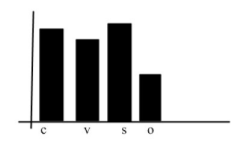 A dot plot is made simply by putting dots above the values  listed on a number line. Dotchart(x)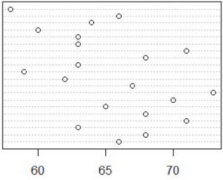 A stem and leaf plot, the data is arranged by values.  o The digits in the largest place are referred to as the  stem and the digits in the smallest place are referred to  as the leaf (leaves).  o The leaves are displayed to the right of the stem.   A split stemplot divides up the stems into equal groups.  o Back-to-back stempots can be used when comparing  two sets of data. Stem(x) 5 | 8, 9 Line 1: 58, 59 6 | 0, 2, 3, 3, 3, 3, 4, 5, 6, 6, 7, 8, 8, 8 Line 2: 60, 62, 63, 63,  63, 63, 647 | 0, 1, 1, 3 Line 3: 65, 66, 66, 67, 68, 68, 68 Stem = tens digit Line 4: 70, 71, 71, 73 Leaf = ones digit  Histograms are created by first dividing the data into  classes, or bins, of equal width.  o Next, count the number of observations in each class.  o The horizontal axis will represent the variable values  and the vertical axis will represent your frequency or  your relative frequency. Hist(x)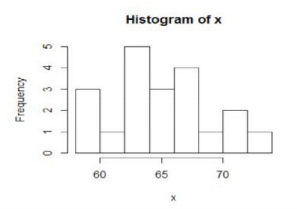 Boxplots not only help identify features about our data  quickly (such as spread and location of center) but can be  very helpful when comparing data sets.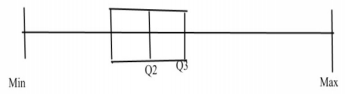o How to make a box plot:  Order the values in the data set in ascending  order (least to greatest).  Find and label the median.  Of the lower half (less than the median—do not  include), find and label Q1.  Of the upper half (greater than the median—do  not include), find and label Q3.  Label the minimum and maximum.  Draw and label the scale on an axis. Plot the five number summary.  Sketch a box starting at Q1 to Q3.  Sketch a segment within the box to represent the  median.  Connect the min and max to the box with line  segments. o Note: If data contains outliers, a box and whiskers  plot can be used instead to display the data.   In a box and whiskers plot, the outliers are  displayed with dots above the value and the  segments begin (or end) at the next data value  within the outlier interval.  A pie chart is a circular chart, divided into sectors, indicating  the proportion of each data value compared to the entire set  of values.  o Pie charts are good for categorical data.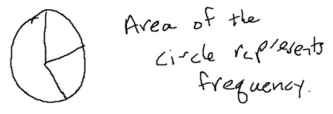 A cumulative frequency plot of the percentages (also called an ogive) can be used to view the total number of events that  occurred up to a certain value. o Example: Here is an ogive for Hudson Auto Repair’s cost of parts sold: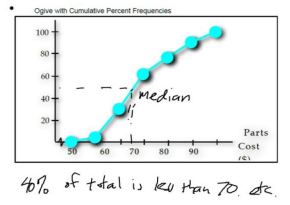∙ Patterns and shapes:- Uniform graphs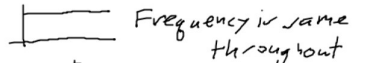- Symmetric graphs- Some other features - Bell Shaped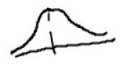- Skewed right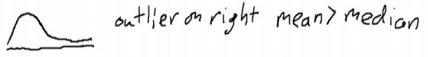- Skewed left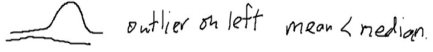Page ExpiredIt looks like your free minutes have expired! Lucky for you we have all the content you need, just sign up here
References: